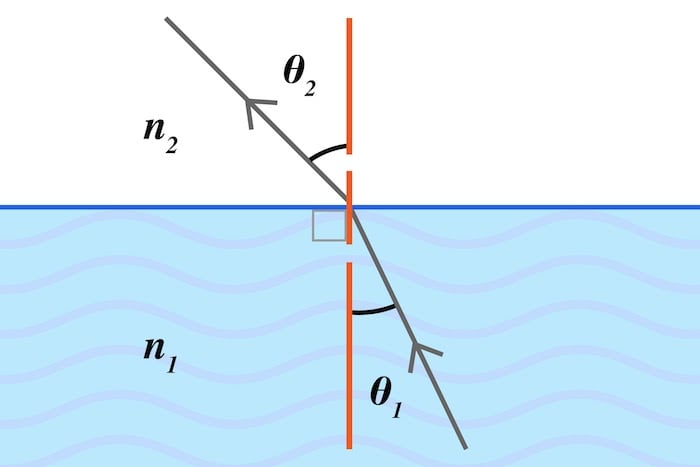# Snell’s Law Calculator

## Given three of the four input parameters (refractive indices, angles of incidence, and refraction) this tool calculates the missing variable based on Snell's law.

### Inputs

This calculator can be applied to the design of optical systems in which it is necessary to understand the behavior and direction of light as it changes mediums of propagation. Depending on the available inputs, this calculator can solve for the refractive index of a medium, the light’s angle of incidence, or its angle of refraction (Figure 1).### Snell's Law Equation:

Snell’s Law is given by the following equation:

$$n_{1}\sin(\theta_{1}) = n_{2}\sin(\theta_{2})$$

Where:

• n1 = Refractive index of the incident medium
• n2 = Refractive index of the refractive medium
• θ1 = Angle of incidence relative to the surface’s normal
• θ2 = Angle of refraction relative to the surface’s normal

The angles are limited to:

$$0 \lt \theta \lt \frac{\pi}{2}$$ radians

or

$$0 \lt \theta \lt 90$$ degrees

##### Table 1. Input limits for the angles of refraction.
Greater Than Less Than Units
0 90 Degrees
0 $$\frac{\pi}{2}$$ (+1.57079) Radians
0 0.25 Turns
0 5400 Minutes of Arc
0 324000 Seconds of Arc

Solving for any of the individual variables requires rearranging the equation accordingly. For example, to solve for θ1 given n1 and n2, the equation can be arranged as follows:

$$\theta_{1} = \sin^{-1}(\frac{n_2\sin(\theta_2)}{\theta_1})$$

The calculator will return “NaN” (meaning Not a Number) if the light would reflect rather than refract for the user-provided refractive indices and angle.